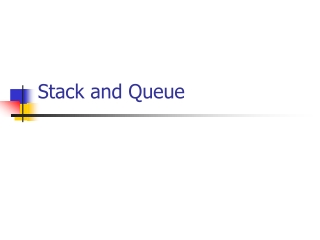DownloadDownload PresentationStack and Queue

# Stack and Queue

Download Presentation## Stack and Queue

- - - - - - - - - - - - - - - - - - - - - - - - - - - E N D - - - - - - - - - - - - - - - - - - - - - - - - - - -
##### Presentation Transcript

1. Stack and Queue

2. Stack From Two Queues • Describe how to implement the stack ADT using two queues • The solution should only use the standard queue operations:ENQUEUE, DEQUEUE, IS-EMPTY • What will be the running timesof the PUSH and POP operations?

3. Stack From Two Queues – Solution • We will call the two queues Q1 and Q2 • Additionally, we will hold a variable called curQueue, which points to the queue that holds the last value pushed to our stack • Initialization: curQueue Q1

4. Stack From Two Queues – Solution (continued) IS-EMPTY return IS-EMPTY (curQueue) PUSH (val) ENQUEUE (curQueue, val) TOP val POP PUSH (val) returnval

5. Stack From Two Queues – Solution (continued) POP otherQueue(curQueue = Q1)? Q2: Q1 while (!IS-EMPTY (curQueue)) temp DEQUEUE (curQueue) if (!IS-EMPTY (curQueue)) // Not last? ENQUEUE (otherQueue, temp) curQueueotherQueue returntemp

6. Stack From Two Queues – Solution Complexity • Since PUSH is simply implemented as a single ENQUEUE operation, its running time is O(1) • For POP operations, we always need to transfer the complete stack contents from one queue to the other, therefore performing a POP takes O(n) time

7. Two Stacks in a Single Array • Describe how to implement two stacks inside a single array • The total number of elements in both stacks is limited by the array length • Therefore, just splitting the array into two equally-sized parts will not do • All stack operations should run in O(1)

8. Two Stacks in a Single Array – Solution Concept • We are given an array A with n elements, numbered 0 to n – 1 • We define two markers t1 and t2that point to the next free cell instacks S1 and S2 respectively • Initialization: • t1 = 0 • t2 = n – 1

9. Two Stacks in a Single Array – Solution Concept • S1 grows up from the beginning of the array, S2 grows down from its end • We know that the array is full when the two markers switch places (t1 > t2) • The complexity is O(1) for all operations • The array usage is optimal

10. Two Stacks in a Single Array – Solution Details S1.IS-EMPTY if (t1 = 0) return true else return false S2.IS-EMPTY if (t2 = n - 1) return true else return false

11. Two Stacks in a Single Array – Solution Details (continued) S1.PUSH (val) if (t1 > t2) error "stack overflow" A[t1] = val t1++ S2.PUSH (val) if (t1 > t2) error "stack overflow" A[t2] = val t2--

12. Two Stacks in a Single Array – Solution Details (continued) S1.POP if (t1 = 0) error "stack underflow" t1-- returnA[t1] S2.POP if (t2 = n - 1) error "stack underflow" t2++ returnA[t2]

13. Two Stacks in a Single Array – Solution Details (continued) S1.TOP if (t1 = 0) error "stack is empty" returnA[t1] S2.TOP if (t2 = n - 1) error "stack is empty" returnA[t2]

14. Evaluating Arithmetic Expressions • Fully parenthesized arithmetic expressions can be evaluated using Dijkstra's two-stack algorithm • Uses two separate stacks – one for the operators and another for the operands • See Java code in: Evaluate.java.html

15. Deque(or Double-Ended Queue) • A deque is a generalization of the Queue ADT, that supports reading and writing from both ends of the queue • We would like to support an input-restricted deque : • Deletion can be made from both ends • Input can only be made at one end

16. Deque • We note that an input-restricted deque is a combination of a queue and a stack • We use a standard queue, and modify it to also support PUSH and POP • The tail (or rear) of the queue will also be used as the stack top

17. Deque • Implementing PUSH is now trivial – simply call ENQUEUE • POP is very similar to DEQUEUE, accept that it returns tail instead of head • The same IS-EMPTY function will work for both the stack and the queue • Check if head = tail

18. Deque ENQUEUE PUSH POP DEQUEUE head(front) tail(rear)